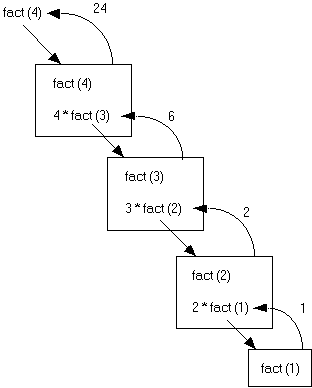CS - C++ Lesson 13:  Recursion

INTRODUCTION:
Recursion is defined as the process of a subprogram calling itself as part of the solution to a problem.  It is a problem solving technique which can turn a long and difficult solution into a compact and elegant answer.  It can also solve problems that are very difficult to solve with a straightforward iterative solution.

The key topics for this lesson are:

A.    Recursion
B.    Pitfalls of Recursion
C.    Recursion Practice

VOCABULARY:       RECURSION                    BASE CASE

DISCUSSION:

A.    Recursion

1.    Recursion occurs when a function calls itself to solve a simpler version of the problem.  With each recursive call, the problem is different from, and simpler than, the original problem.

2.    Recursion involves the internal use of a stack.  A stack is a data abstraction which works like this:  New data is "pushed," or added to the top of the stack.  When information is removed from the stack it is "popped," or removed from the top of the stack.  The recursive calls of a function will be stored on a stack, and manipulated in a similar manner.

3.    The problem of solving factorials is our first example of recursion.  The factorial operation in mathematics is illustrated below.

1! = 1

2! = 2 * 1                or          2 * 1!

3! = 3 * 2 * 1          or          3 * 2!

4! = 4 * 3 * 2 *1     or          4 * 3!

Notice that each successive line can be solved in terms of the previous line.  For example, 4! is equivalent to the problem

4 * 3!.

On the next page you will find a recursive function to solve the factorial problem.  Notice in the last line of the function the recursive call.  The function calls another implementation of itself to solve a smaller version of the problem.

 int  fact (int n) //  returns the value of n! //  precondition:  n >= 1 {          if (1 == n)  return 1;          else  return  n * fact(n - 1); }

4.    The base case is a fundamental situation where no further problem solving is necessary.  In the case of finding factorials, the answer of 1! is by definition = 1.  No further work is needed.

5.    Suppose we call the function to solve fact(4).  This will result in four calls of function fact.

fact(4):  This is not the base case (1 == n), so we return the result of

4 * fact(3).  This multiplication will be solved until an answer is found for fact (3).  This leads to the second call of fact to solve fact(3).

fact(3):  Again, this is not the base case and we return 3 * fact (2).

This leads to another recursive call to solve fact(2).

fact(2):  Still, this is not the base case, we solve 2 * fact(1).

fact(1):  Finally we reach the base case which returns the value 1.

6.    When a recursive call is made, the current computation is temporarily suspended and placed on the stack with all its current information available for later use.

7.    A completely new copy of the function is used to evaluate the recursive call.  When that is completed, the value returned by the recursive call is used to complete the suspended computation.  The suspended computation is removed from the stack and its work now proceeds.

8.    When the base case is encountered the recursion will now unwind and result in a final answer.  The expressions below should be read from right to left.

fact (4) = 4 * fact (3) = 3 * fact (2) = 2 * fact (1) = 1

24 <-- 4 * 6 <-- 3 * 2 <-- 2 * 1

Here is a picture.   Look at what happens:Each box represent a call of function fact.  To solve fact (4) requires four calls of function fact.

9.    Notice that when the recursive calls were made inside the else statement, the value fed to the recursive call was (n-1).  This is where the problem is getting smaller and simpler with the eventual goal of solving 1!.

B.    Pitfalls of Recursion

1.    If the recursion never reaches the base case, the recursive calls will continue until the computer runs out of memory and the program crashes.  Experienced programmers try to examine the remains of a crash.  The message “stack overflow error” or “heap storage exhaustion” indicates a possible runaway recursion.

2.    When programming recursively, you need to make sure that the algorithm is moving toward the base case.  Each successive call of the algorithm must be solving a simpler version of the problem.

3.    Any recursive algorithm can be implemented iteratively, but sometimes only with great difficulty.  However, a recursive solution will always run more slowly than an iterative one because of the overhead of opening and closing the recursive calls.

C.    Recursion Practice

1.    Write a recursive power function that raises a base to some exponent, n.  We will use integers to keep things simple.

2.

double  power (int  base, int n)
//  Recursively determines base raised to the nth power.
//  Assumes 0 <= n <= 10.

SUMMARY/REVIEW:          Recursion takes some time and practice to get used to.  Eventually you want to be able to think recursively without the aid of props and handouts.  Study the examples provided in these notes and work it through for yourself.  Recursion is a very powerful programming tool for solving difficult problems.

ASSIGNMENT:                     Lab Exercise L.A.13.1, Fibonacci